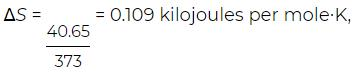Courses

# Enthalpy and the heat of reaction Physics Notes | EduRev

## Basic Physics for IIT JAM

Created by: Akhilesh Thakur

## Physics : Enthalpy and the heat of reaction Physics Notes | EduRev

The document Enthalpy and the heat of reaction Physics Notes | EduRev is a part of the Physics Course Basic Physics for IIT JAM.
All you need of Physics at this link: Physics

As discussed above, the free energy change Wmax = −ΔG corresponds to the maximum possible useful work that can be extracted from a reaction, such as in an electrochemical battery. This represents one extreme limit of a continuous range of possibilities. At the other extreme, for example, battery terminals can be connected directly by a wire and the reaction allowed to proceed freely without doing any useful work. In this case W′ = 0, and the first law of thermodynamics for the reaction becomes
ΔU = Q0 − PΔV,

where Q0 is the heat absorbed when the reaction does no useful work and, as before, PΔV is the atmospheric work term. The key point is that the quantities ΔU and PΔV are exactly the same as in the other limiting case, in which the reaction does maximum work. This follows because these quantities are state functions, which depend only on the initial and final states of a system and not on any path connecting the states. The amount of useful work done just represents different paths connecting the same initial and final states. This leads to the definition of enthalpy (H), or heat content, as
H = U + PV.
Its significance is that, for a reaction occurring freely (i.e., doing no useful work) at constant temperature and pressure, the heat absorbed is
Q0 = ΔU + PΔV = ΔH,

where ΔH is called the heat of reaction. The heat of reaction is easy to measure because it simply represents the amount of heat that is given off if the reactants are mixed together in a beaker and allowed to react freely without doing any useful work.
The above definition for enthalpy and its physical significance allow the equation for ΔG to be written in the particularly illuminating and instructive form.
ΔG = ΔH − TΔS.

Both terms on the right-hand side represent heats of reaction but under different sets of circumstances. ΔH is the heat of reaction (i.e., the amount of heat absorbed from the surroundings in order to hold the temperature constant) when the reaction does no useful work, and TΔS is the heat of reaction when the reaction does maximum useful work in an electrochemical cell. The (negative) difference between these two heats is exactly the maximum useful work −ΔG that can be extracted from the reaction. Thus, useful work can be obtained by contriving for a system to extract additional heat from the environment and convert it into work. The difference ΔHTΔS represents the fundamental limitation imposed by the second law of thermodynamics on how much additional heat can be extracted from the environment and converted into useful work for a given reaction mechanism. An electrochemical cell (such as a car battery) is a contrivance by means of which a reaction can be made to do the maximum possible work against an opposing electromotive force, and hence the reaction literally becomes reversible in the sense that a slight increase in the opposing voltage will cause the direction of the reaction to reverse and the cell to start charging up instead of discharging.
As a simple example, consider a reaction in which water turns reversibly into steam by boiling. To make the reaction reversible, suppose that the mixture of water and steam is contained in a cylinder with a movable piston and held at the boiling point of 373 K (100 °C) at 1 atmosphere pressure by a heat reservoir. The enthalpy change is ΔH = 40.65 kilo joules per mole, which is the latent heat of vaporization. The entropy change isrepresenting the higher degree of disorder when water evaporates and turns to steam. The Gibbs free energy change is ΔG = ΔH − TΔS. In this case the Gibbs free energy change is zero because the water and steam are in equilibrium, and no useful work can be extracted from the system (other than work done against the atmosphere). In other words, the Gibbs free energy per molecule of water (also called the chemical potential) is the same for both liquid water and steam, and so water molecules can pass freely from one phase to the other with no change in the total free energy of the system.

Offer running on EduRev: Apply code STAYHOME200 to get INR 200 off on our premium plan EduRev Infinity!

## Basic Physics for IIT JAM

109 videos|157 docs|94 tests

,

,

,

,

,

,

,

,

,

,

,

,

,

,

,

,

,

,

,

,

,

;